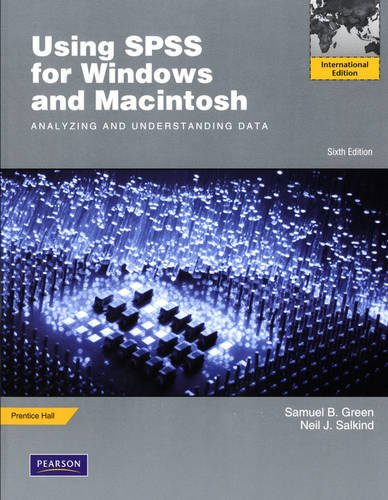### Using SPSS for Windows and Macintosh:Analyzing and Understanding Data:International Edition

 Yazar : Samuel B. Green ; Neil J. Salkind Yayınevi : Pearson Education ISBN : 9780205670307 Baskı yılı : 2011 Sayfa sayısı : 480 Ağırlık : 0,90 kg Edisyon : 6 Stok durumu : Tükendi

For courses in Introductory Statistics and Research Methods courses using SPSS.

?

Using SPSS guides students through the most basic of SPSS techniques using?step-by-step descriptions, presents statistical techniques and?instruction on how to conduct statistical analyses, and explains in detail how to avoid all the?obstacles common in the study of statistics.

?

The development of easy-to-use statistical software like SPSS has changed the way statistics is being taught and learned. Students can make transformations of variables, create?graphs of distributions of variables,?and select among statistical analyses all at the click of a button. However, even with these advancements,?students sometimes still find statistics to be an arduous task. They?continue to be?faced with many potential obstacles and they might feel overwhelmed and stressed rather than challenged and excited about the potential for mastering these important skills. Samuel Green and Neil Salkind have written a text to specifically address these issues.

Preface Part I Introducing SPSS Unit 1 Getting Started with SPSS Lesson 1 Starting SPSS Lesson 2 The SPSS Main Menus and Toolbar Lesson 3 Using SPSS Help Lesson 4 A Brief SPSS Tour Unit 2 Creating and Working with Data Files Lesson 5 Defining Variables Lesson 6 Entering and Editing Data Lesson 7 Inserting and Deleting Cases and Variables Lesson 8 Selecting, Copying, Cutting, and Pasting Data Lesson 9 Printing and Exiting an SPSS Data File Lesson 10 Exporting and Importing SPSS Data Lesson 11 Validating SPSS Data Unit 3 Working with Data Lesson 12 Finding Values, Variables, and Cases Lesson 13 Recoding Data and Computing Values Lesson 14 Sorting, Transposing, and Ranking Data Lesson 15 Splitting and Merging Files Unit 4A Working with SPSS Charts and Output for Windows Lesson 16A Creating an SPSS Chart Lesson 17A Enhancing SPSS Charts Lesson 18A Using the Viewer and Pivot Tables Unit 4B Working with SPSS Charts and Output for Macintosh Lesson 16B Creating an SPSS Chart Lesson 17B Enhancing SPSS Charts Lesson 18B Using the Viewer and Pivot Tables Part II Working with SPSS Procedures Unit 5 Creating Variables and Computing Descriptive Statistics Lesson 19 Creating Variables Lesson 20 Univariate Descriptive Statistics for Qualitative Variables Lesson 21 Univariate Descriptive Statistics for Quantitative Variables Unit 6 t Test Procedures Lesson 22 One-Sample t Test Lesson 23 Paired-Samples t Test Lesson 24 Independent-Samples t Test Unit 7 Univariate and Multivariate Analysis-of-Variance Techniques Lesson 25 One-Way Analysis of Variance Lesson 26 Two-Way Analysis of Variance Lesson 27 One-Way Analysis of Covariance Lesson 28 One-Way Multivariate Analysis of Variance Lesson 29 One-Way Repeated-Measures Analysis of Variance Lesson 30 Two-Way Repeated-Measures Analysis of Variance Unit 8 Correlation, Regression, and Discriminant Analysis Procedures Lesson 31 Pearson Product-Moment Correlation Coefficient Lesson 32 Partial Correlations Lesson 33 Bivariate Linear Regression Lesson 34 Multiple Linear Regression Lesson 35 Discriminant Analysis Unit 9 Scaling Procedures Lesson 36 Factor Analysis Lesson 37 Internal Consistency Estimates of Reliability Lesson 38 Item Analysis Using the Reliability Procedure Unit 10 Nonparametric Procedures Lesson 39 Binomial Test Lesson 40 One-Sample Chi-Square Test Lesson 41 Two-Way Contingency Table Analysis Using Crosstabs Lesson 42 Two Independent-Samples Test: The Mann-Whitney U Test Lesson 43 K Independent-Samples Tests: The Kruskal-Wallis and the Median Tests Lesson 44 Two Related-Samples Tests: The McNemar, the Sign, and the Wilcoxon Tests Lesson 45 K Related-Samples Tests: The Friedman and the Cochran Tests Appendix A Data for Crab Scale and Teacher Scale Appendix B Methods for Controlling Type I Error across Multiple Hypothesis Tests Appendix C Selected Answers to Lesson Exercises References Index